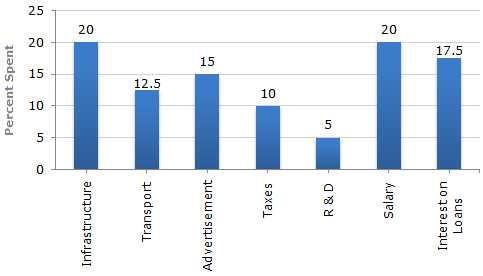# Data Interpretation - Bar Charts

Directions to Solve

The bar graph given below shows the percentage distribution of the total expenditures of a company under various expense heads during 2003.

Percentage Distribution of Total Expenditure of a Company1.
The total amount of expenditures of the company is how many times of expenditure on research and development?
27
20
18
8
Explanation:

Let the total expenditures be Rs. x.

Then, the expenditure on Research and Development (R & D)

= Rs. (5% of x)

 = Rs.5 x x100

 = Rs.x. 20Ratio of the total expenditure to the expenditure on R & D

 =xx/20

 =20. 1

Then, the total expenditure is 20 times the expenditure of Research and Development.

2.
If the expenditure on advertisement is 2.10 crores then the difference between the expenditure on transport and taxes is?
Rs. 1.25 crores
Rs. 95 lakhs
Rs. 65 lakhs
Rs. 35 lakhs
Explanation:

Let the total expenditure be Rs. x crores.

 Then, 15% of x = 2.10x =2.10 x 100= 14. 15Total expenditure = Rs. 14 crores

and so, the difference between the expenditures on transport and taxes

= Rs. [(12.5 - 10)% of 14] crores

= Rs. [2.5% of 14] crores

= Rs. 0.35 crores

= Rs. 35 lakhs

3.
What is the ratio of the total expenditure on infrastructure and transport to the total expenditure on taxes and interest on loans?
5:4
8:7
9:7
13:11
Explanation:

Let the total amount of expenditures be Rs. x.

Then, the total expenditure on infrastruture and transport

= Rs. [(20 + 12.5)% of x]

= Rs. [32.5% of x]

 = Rs.32.5x100

and total expenditure on taxes and interest on loans

= Rs. [(10 + 17.5)% of x]

= Rs. [27.5% of x]

 = Rs.27.5x100Required ratio =32.5x/100= 13 . 27.5x/100 11

4.
If the interest on loans amounted to Rs. 2.45 crores then the total amount of expenditure on advertisement, taxes and research and development is?
Rs. 7 crores
Rs. 5.4 crores
Rs. 4.2 crores
Rs. 3 crores
Explanation:

Let the total expenditure be Rs. x crores.

Then, 17.5% of x = 2.45x = 14.Total expenditure = Rs. 14 crores.

and so, the total expenditure on advertisement, taxes and Research and Development

= Rs. [(15 + 10 + 5)% of 14] crores

= Rs. [30% of 14] crores

= Rs. 4.2 crores.

5.
The expenditure on the interest on loans is by what percent more than the expenditure on transport?
5%
10%
20%
40%
 Then, the expenditure on interest on loans = Rs. (17.5% of x) = Rs.17.5 x100
 and the expenditure on transport = Rs. (12.5% of x) = Rs.12.5 x. 100Difference between the two expenditures
 = Rs.17.5x - 12.5x100 100
 = Rs.5x100
 and so, the required percentage =5x/100 x 100% = 40%. 12.5x/100